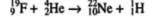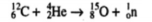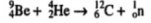Reactions involving α-particles

 Reaction: Verification: F199 + He42 → Ne2210 + p11 438 + 90 → 516 + 12 528=528Reaction: Verification: C126 + He42 → O158 + n10 270 + 90 → 327 + 33 360=360Reaction: Verification: U23892 + He42 → Pu24194 + n10 5922 + 90 → 5979 + 33 6012=6012Reaction: Verification: Be94 + He42 → C126 + n10 213 + 90 → 270 + 33 2163=2163References: Sharma B. K. Nuclear and Radiation Chemistry. Krishna Prakashan Media, 2001. P. 106.